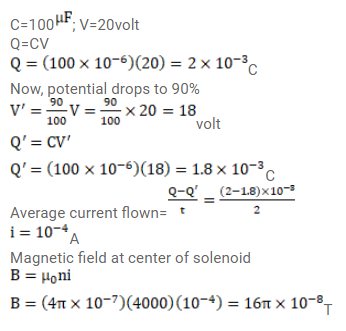# A capacitor of capacitance 100mf is connected to a battery of

Question:

A capacitor of capacitance $100^{\mu \mathrm{F}}$ is connected to a battery of $20 v o l t$ for a long time and then disconnected from it. It is now connected across a long solenoid having 4000 turns per meter. It is found that the potential difference across the capacitor drops to $90 \%$ of its maximum value in $2.0$ seconds. Estimate the average the magnetic field produced at the center of the solenoid during this period.

Solution: